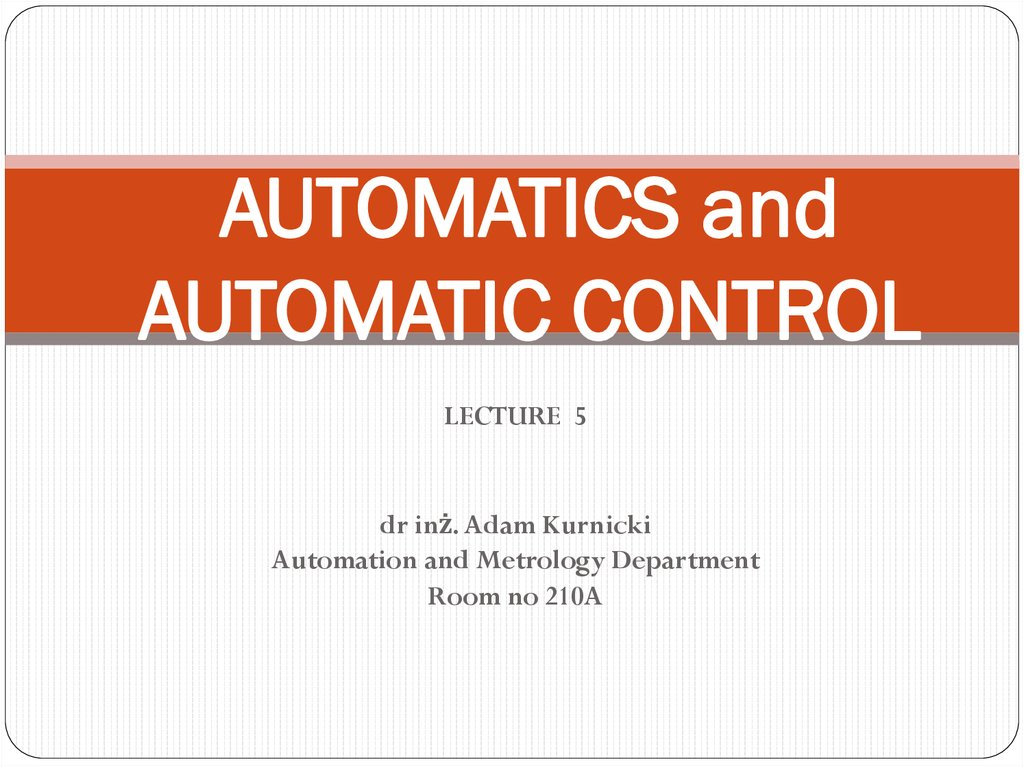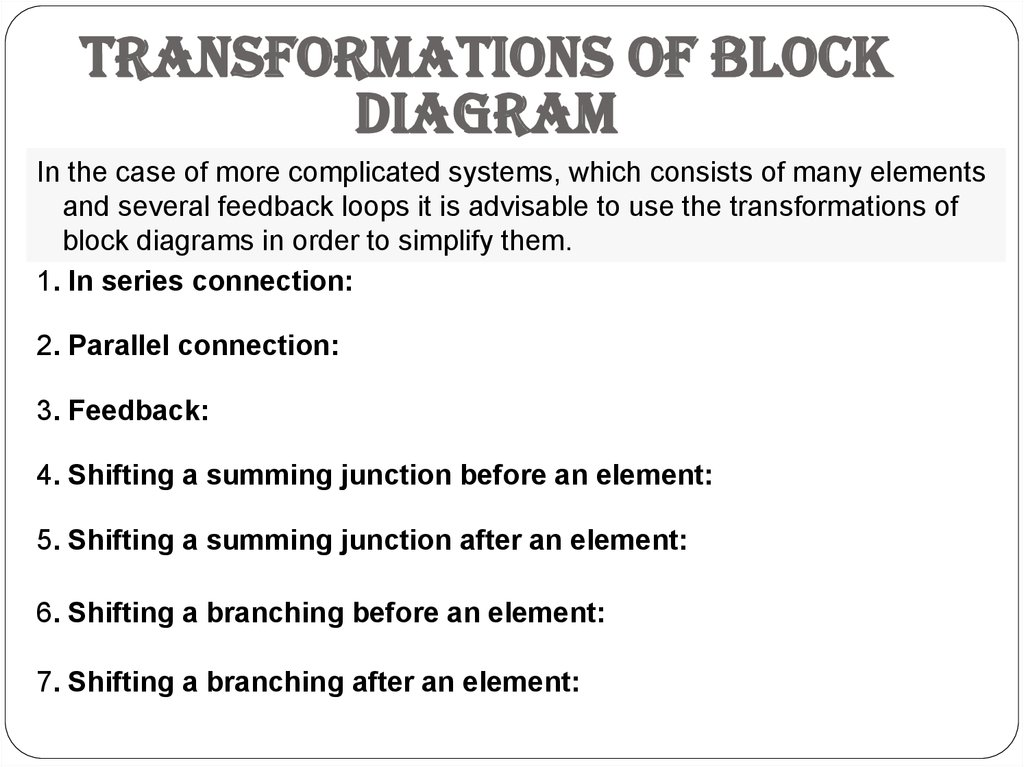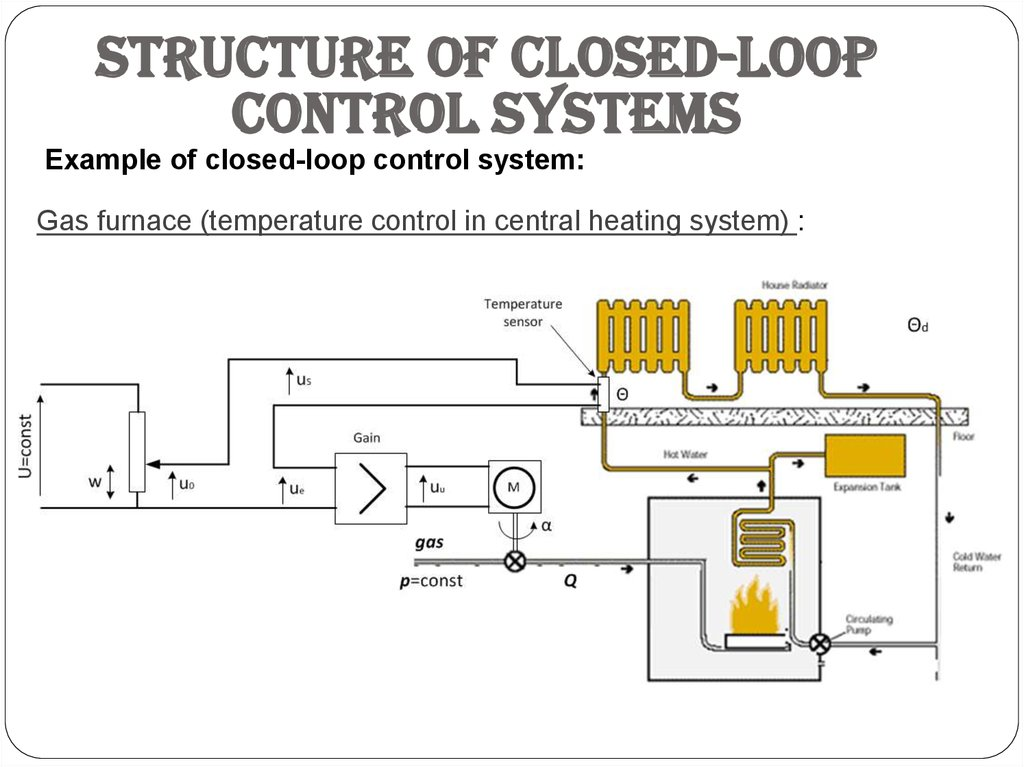Transformations of block diagram

1. AUTOMATICS and AUTOMATIC CONTROL

LECTURE 5
Automation and Metrology Department
Room no 210A

2.

Transformations of block
diagram
In the case of more complicated systems, which consists of many elements
and several feedback loops it is advisable to use the transformations of
block diagrams in order to simplify them.
1. In series connection:
2. Parallel connection:
3. Feedback:
4. Shifting a summing junction before an element:
5. Shifting a summing junction after an element:
6. Shifting a branching before an element:
7. Shifting a branching after an element:

3.

Structure of closed-loop
control systems
Example of closed-loop control system:
Gas furnace (temperature control in central heating system) :

4.

Structure of closed-loop
control systems
Example of closed-loop control system:
Gas furnace (block diagram) :

5.

Structure of closed-loop
control systems
Closed-loop control system (general block diagram):
yo (t) – reference (set point, input signal)
y(t) – controlled signal (output signal)
ys (t) – measured controlled signal (ys (t) ≈ y(t))
e(t) - error e(t) = yo (t) - ys (t)
u(t) – control signal
d(t) - disturbance

6.

Structure of closed-loop
control systems
Closed-loop control system (general equations):
Y ( s) Gr (s) Go (s) E ( s) D( s) Gd (s)
E (s) Y0 (s) YS (s) Y0 (s) Y (s)GS (s)
Y (s) Gr (s) Go (s) Y0 (s) Y (s)GS (s) D(s) Gd (s)
Gr ( s)Go ( s )
Gd ( s)
Y (s)
Y0 ( s )
D( s )
1 Gr ( s)Go ( s )GS ( s)
1 Gr ( s)Go ( s)GS ( s )
E (s) Y0 (s) Gr (s) Go (s) E (s) D( s) Gd (s) GS (s)
Gd ( s )Gs ( s )
1
E ( s)
Y0 ( s )
D( s)
1 Gr ( s )Go ( s)GS ( s )
1 Gr ( s )Go ( s )GS ( s )

7.

Structure of closed-loop
control systems
Output-reference response transfer function:
G ( s)
Y (s)
Y0 ( s)
Gr ( s)Go ( s)
D( s) 0 1 G ( s)G ( s)G ( s)
r
o
S
Output-disturbance response transfer function:
GD ( s )
Y ( s)
D( s )
Gd ( s)
Y0 ( s ) 0 1 G ( s)G ( s)G ( s)
r
o
S
Error-reference response transfer function:
GE ( s )
E ( s)
Y0 ( s)
1
D( s) 0 1 G ( s)G ( s)G ( s)
r
o
S